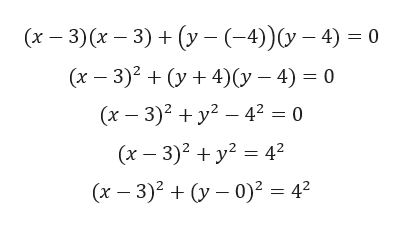# Find the center-radius form of the circle described. A circle having a diameter with end points (3, -4) and (3, 4)

Question
1 views

Find the center-radius form of the circle described. A circle having a diameter with end points (3, -4) and (3, 4)

check_circle

Step 1

Given, A circle having a diameter with end points (3, -4) and (3, 4).

We know that,  the equation of the circle when end points of the diameter are

(x1, y1) and (x2, y2) is given by

Step 2

Since, circle having a diameter with ...help_outlineImage Transcriptionclose(r-3) ( 3)y - (4)40 (x 3)2y4)(y -4) 0 (x 3)2y 42 = 0 (x-3)2 y 42 (x 3)2y0)2 42 fullscreen

### Want to see the full answer?

See Solution

#### Want to see this answer and more?

Solutions are written by subject experts who are available 24/7. Questions are typically answered within 1 hour.*

See Solution
*Response times may vary by subject and question.
Tagged in

### Other